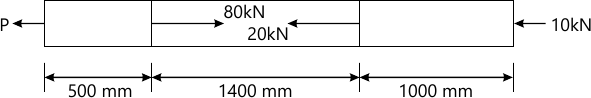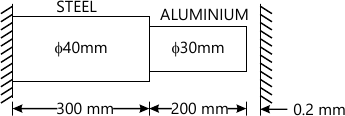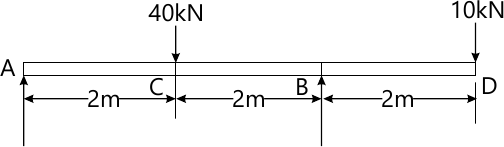MORE IN Mechanics of Materials
VTU Mechanical Engineering (Semester 3)
Mechanics of Materials
December 2015
Total marks: --
Total time: --
INSTRUCTIONS
(1) Assume appropriate data and state your reasons
(2) Marks are given to the right of every question
(3) Draw neat diagrams wherever necessary

1 (a) Define (i) Proof stress (ii) Proportionality limit (iii) Principle of superposition (iv) Hooke's law.
4 M
1 (b) Derive an expression for the total elongation of the tapered bar varying diameter from d1, to d2, when subjected to axial load P.
8 M
1 (c) A brass bar having of uniform cross ? sectional area of 300mm2 is subjected to a load as shown below. Find the total elongation of bar and the magnitude of load 'P' if Young's modulus is 84 GPa.8 M

2 (a) Define: (i) Poisson's ratio (ii) Modular ratio.
2 M
2 (b) Establish relationship between Young's modulus and rigidity modulus.
8 M
2 (c) For the stepped bar shown in fig. Q2(c), what is the maximum temperature rise which will not produce stress in the bar. Also find the stress induced when the temperature rise is 40°C. Take ES=200GPa; EA=100GPa; as=12×10-6/°C; aA 18×10-6/°C.10 M

3 (a) Define: (i) Plant stress (ii) Principle strain
2 M
3 (b) Derive the expression for normal stress, shear stress and resultant stress on oblique plane inclined at an angle 'θ' with vertical axis in a biaxial direct stress system.
8 M
3 (c) At a certain point in a strained material the values of normal stresses across two planes at right angles to each other are 80MPa and 32 Mpa, both are tensile and there is a shear stress of 32 Mpa clockwise on the plane carrying 80MPa stress. Determine; principle stresses, maximum shear stress and their planes.
10 M

4 (a) Determine the strain energy in cantilever beam of uniform cross ? section and length 'I' subjected to a uniformaly distributed load of 'W' kN/m over the entire span.
4 M
4 (b) For a thin cylindrical shell, the L/d ratio is 3 and its initial volume is 20m3. The ultimate stress for the cylinder material is 200MPa. Determine the wall thickness. If it has to convey water under a head of 200m. Take F.O.S. as 2.
8 M
4 (c) Calculate the maximum external to internal ratio for a thick cylinder with internal fluid pressure of 15MPa and maximum hoop stress is 60MPa.
8 M

5 (a) Deduce the relationship between relating load (W), shear force (F) and bending moment (M).
6 M
5 (b) A beam ABCD is simply supported at B and C, 4.5m apart and overhanging parts AB and CD are 1.5m and 2m long respectively. The beam carries a uniformly distributed load of 10kN/m between A&C. There is a clock wise couple of 60kN-m at D. Then draw S.F. and B.M. diagram and mark salient points.
14 M

6 (a) Enumerate the assumptions made in theory of pure bending. Write the bending equation with usual notations.
6 M
6 (b) A beam of an 1- Section consists of 180mm × 15mm flanges and a web a 280mm × 15mm thickness. It is subjected to a bending moment of 120 kN-m. Sketch the bending stress distribution along the depth of the section.
6 M
6 (c) Prove that in case of a rectangular section of a beam the maximum shear stress is 1.5 times average shear stress.
8 M

7 (a) Derive an expression $$\text{EI} \dfrac {d^2y}{dx^2}=\text M,$$ with usual notations.
10 M
7 (b) Determine the deflection under the loads in the beam shown in fig Q7(b). Take Flexural rigidity as EI, through.10 M

8 (a) A hollow circular steel shaft has to transmit 60KW at 210 rpm such that the maximum shear stress does not exceed 60MN/m2. If the ratio of internal to external diameter is equal to 3/4 and the value of rigidity modulus is 84GPa, find the dimensions of the shaft and angle of twist in length of 3m.
10 M
8 (b) Derive an expression for the critical load in a column subjected to compressive load, when both ends are fixed.
10 M

More question papers from Mechanics of Materials